# Answer Key 10.4

1.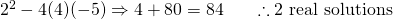2.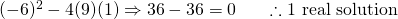3.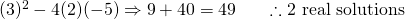4.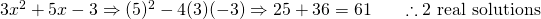5.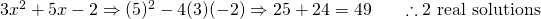6.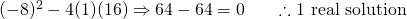7.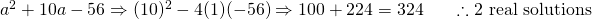8.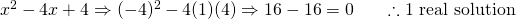9.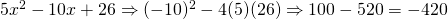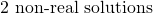10.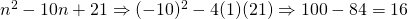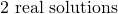1.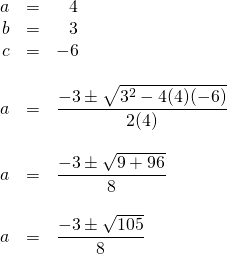2.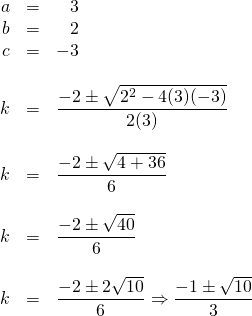3.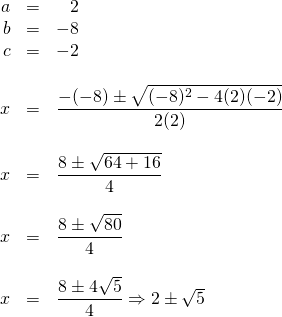4.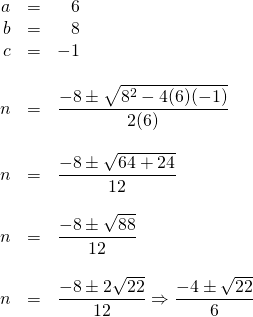5.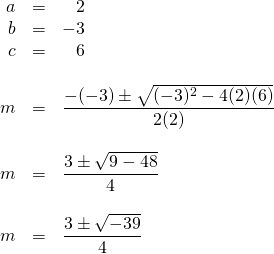A negative square root means there are 2 non-real solutions or no real solution.

6.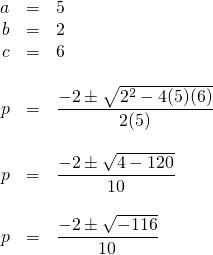A negative square root means there are 2 non-real solutions or no real solution.

7.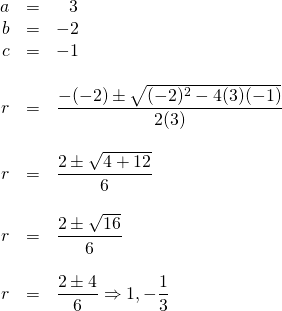8.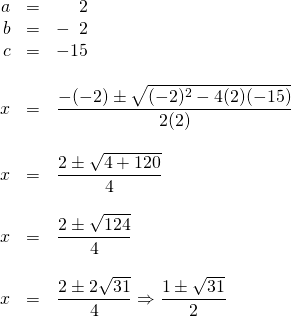9.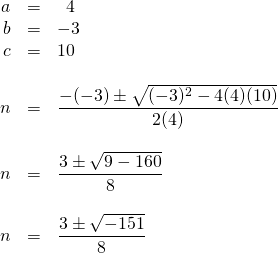∴ 2 non-real solutions

10.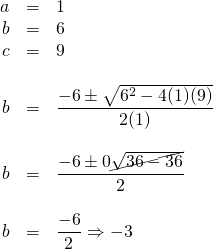11.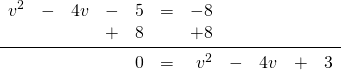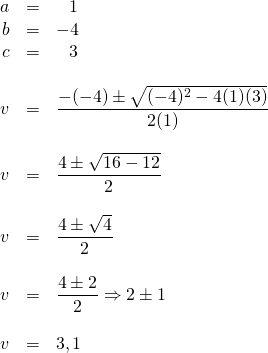12.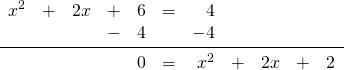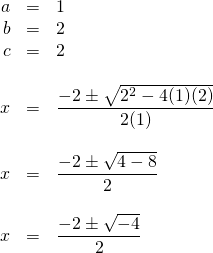∴ 2 non-real solutions